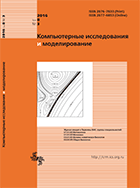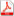Issue 3, 2016 Vol. 8

# All issues

Stability investigation of finite-difference schemes of lattice Boltzmann method for diffusion modellingpdf (4604K)  / List of references

Stability of finite difference schemes of lattice Boltzmann method for modelling of 1D diffusion for cases of D1Q2 and D1Q3 lattices is investigated. Finite difference schemes are constructed for the system of linear Bhatnagar–Gross–Krook (BGK) kinetic equations on single particle distribution functions. Brief review of articles of other authors is realized. With application of multiscale expansion by Chapman–Enskog method it is demonstrated that system of BGK kinetic equations at small Knudsen number is transformated to scalar linear diffusion equation. The solution of linear diffusion equation is obtained as a sum of single particle distribution functions. The method of linear travelling wave propagation is used to show the unconditional asymptotic stability of the solution of Cauchy problem for the system of BGK equations at all values of relaxation time. Stability of the scheme for D1Q2 lattice is demonstrated by the method of differential approximation. Stability condition is written in form of the inequality on values of relaxation time. The possibility of the reduction of stability analysis of the schemes for BGK equations to the analysis of special schemes for diffusion equation for the case of D1Q3 lattice is investigated. Numerical stability investigation is realized by von Neumann method. Absolute values of the eigenvalues of the transition matrix are investigated in parameter space of the schemes. It is demonstrated that in wide range of the parameters changing the values of modulas of eigenvalues are lower than unity, so the scheme is stable with respect to initial conditions.

Keywords: lattice Boltzmann method, stability
Citation in English: Krivovichev G.V. Stability investigation of finite-difference schemes of lattice Boltzmann method for diffusion modelling // Computer Research and Modeling, 2016, vol. 8, no. 3, pp. 485-500
Citation in English: Krivovichev G.V. Stability investigation of finite-difference schemes of lattice Boltzmann method for diffusion modelling // Computer Research and Modeling, 2016, vol. 8, no. 3, pp. 485-500
DOI: 10.20537/2076-7633-2016-8-3-485-500
Views (last year): 2. Citations: 1 (RSCI).

Full-text version of the journal is also available on the web site of the scientific electronic library eLIBRARY.RU

The journal is included in the Russian Science Citation Index

The journal is included in the List of Russian peer-reviewed journals publishing the main research results of PhD and doctoral dissertations.

International Interdisciplinary Conference "Mathematics. Computing. Education"

The journal is included in the RSCI

Indexed in Scopus